## How to insert formula to sum a column or row of table in Word?

You may know how to sum or calculate data in Excel, but do you know how to sum the data in a column or row of a table in Word document? Let’s talk about the methods of calculate the data in a table in Word.

Sum a column of a table in Word

Sum a row of a table in Word

Do other calculation in a table in Word

####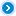Sum a column of a table in Word

###### Amazing! Use Efficient Tabs in Word (Office) like Chrome, Firefox and New Internet Explorer!

If you want to sum a column data of the table shown as below, you can do as follow steps: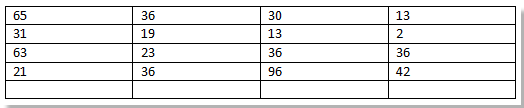Step 1. Place the cursor at the blank cell of the first column, then click layout > Formula, see screenshot: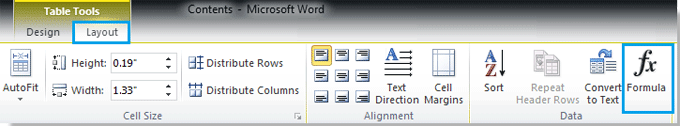Step 2. A dialog named Formula is displayed, typing =SUM(ABOVE) in the Formula text box. See screenshot: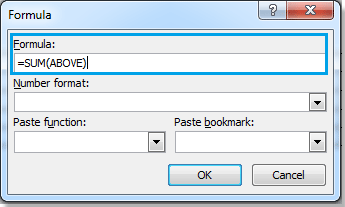Step 3. Click OK. Then the above data are added together and the result is shown in the blank cell. See screenshot: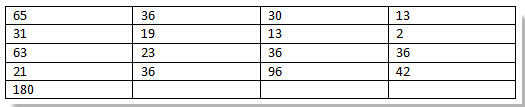Tip: To sum the same column data below the blank cell, please type =SUM(BELOW) in the Formula text box.

####Sum a row of a table in Word

If you want to sum a row of a table in a Word document, do as follow:

Step 1. Place the cursor at the blank cell of the first row, then click layout > Formula, see screenshot:Step 2. A dialog named Formula is displayed, typing =SUM(LEFT) in the formula text box. See screenshot: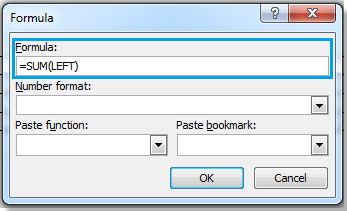Step 3. Click OK. Then all the left data of the first row are added together and the result is shown in the blank cell. See screenshot: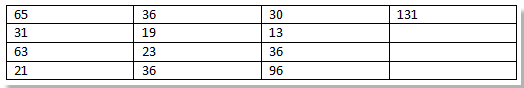Tip: To sum the same row data on the right of the blank cell, please type =SUM(RIGHT) in the Formula text box.

####Do other calculation in a table in Word

In Word, you can do other calculations in table such as average, production and so on.

Step 1. Click the blank cell you want to show the calculated result, then click layout > Formula.Step 2. A dialog named Formula is displayed, typing "=" in the formula text box and select the function you want to apply from the Paste function list. In this case, I select Max. See screenshots: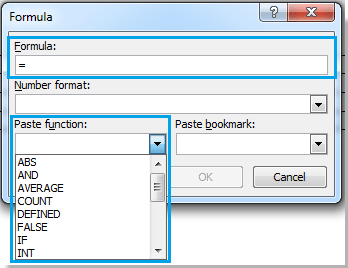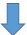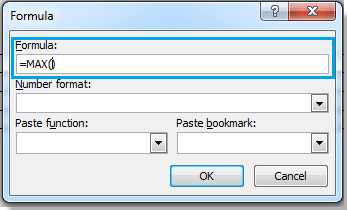Step 3. Between the parenthesis in the Formula text box, please do as follows:

• Typing ABOVE to calculate all the data above the blank cell.
• Typing BELOW to calculate all the data below the blank cell.
• Typing LEFT to calculate all the data on the left of the blank cell.
• Typing RIGHT to calculate all the data on the right of the blank cell.

In this case, I Typing LEFT, see screenshot: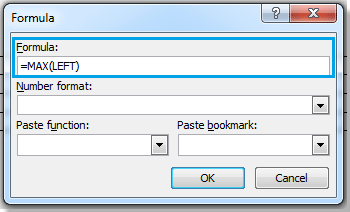Step 4. Click OK, you can see the result shown as below: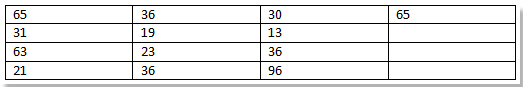### Recommended Word Productivity Tools

#### Kutools For Word - More Than 100 Advanced Features For Word, Save Your 50% Time

• Complicated and repeated operations can be done one-time processing in seconds.
• Insert multiple images across folders into Word document at once.
• Merge and combine multiple Word files across folders into one with your desired order.
• Split the current document into separate documents according to heading, section break or other criteria.
• Convert files between Doc and Docx, Docx and PDF, collection of tools for common conversions and selection, and so on...
No ratings yet. Be the first to rate!
This comment was minimized by the moderator on the site
I am trying to add together the C3 through S3. It says Syntax Error. So....?
This comment was minimized by the moderator on the site
What if I want to, say multiply the number in column 3 by the number in column 4 on the row?
This comment was minimized by the moderator on the site
There are 5 cells going across "Total" and the number, a second "Total" and the number and "Total" where the sum of both should be. Sum left is only pulling the number to the left and not adding the other numbers in the row. I used the formula =SUM(LEFT). Does it not work when there is text? How can I make this work?
This comment was minimized by the moderator on the site
I was looking for a similar solution and didn't find one. However, I found a work around. At the end of the text string, I included a zero and change the color the font to white. This allowed my formula to calculate across rows that had text in them. This is a fix for a very basic table. Hope this helps.
This comment was minimized by the moderator on the site
I need the answer to this one too...did you find it? There are blank cells between the amounts you want to sum. I think you can just put a zero as a placeholder in the blank cells
This comment was minimized by the moderator on the site
how to get 5 X 10 = 50 this but both a in same raw but different column between them have UNIT column so how to solve this issue?
This comment was minimized by the moderator on the site

You can achieve this with:
PRODUCT(LEFT)
Then it will multiply every column on the left side of the calculation field column.
This comment was minimized by the moderator on the site
how to sum table in Word
This comment was minimized by the moderator on the site
To sum the columns above you need to make sure that no row is empty and use:
SUM(ABOVE)
This comment was minimized by the moderator on the site
could you explain me how can i prepare MIS in Ms Word file can u send the sample file in my email id
This comment was minimized by the moderator on the site
Does it recalculate when the numbers change?
This comment was minimized by the moderator on the site
Click F9 key to recalculate the cell
This comment was minimized by the moderator on the site
HI, When I use the above formula the formula is displayed in the cell but the it is not calculating, please let me know if we need to to any setup changes
This comment was minimized by the moderator on the site
could you explain me how can i prepare MIS in Ms Word file can u send the sample file in my email id
This comment was minimized by the moderator on the site
how to sum table in Word
This comment was minimized by the moderator on the site
HI am creating a document in a word format, but I need to put some figures and add them, are there simple way to create an addition formula in it? thanks for the help
This comment was minimized by the moderator on the site
I Have a row of numbers.. i want to plus 40% on all of them to save going through and manually doing it myself. any thought??
This comment was minimized by the moderator on the site
hello could you explain me how shoul i sum many numbers in a table in which i got"-" dashes instead of "0"? i would really apprciate. best regards.
This comment was minimized by the moderator on the site
Hi, could you explain me how shoul I sum many numbers in a table in which I got "-" dashes instead of "0"? I would really appreciate. Best regards, Riccardo Nicolai
This comment was minimized by the moderator on the site
Really Really appreciate your ways of explanations. Anyways thanks a lot!!
There are no comments posted here yet